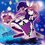This inequality problem is from Phan Boi Chau High School for the Gifted Grade 10 Selection Test, Nghe An, Vietnam (Yes I'm very bad at inequalities):

Let $a,b,c$ be positive reals. Find the minimum value of $P=\dfrac{a^2}{(a+b)^2}+\dfrac{b^2}{(b+c)^2}+\dfrac{c}{4a}$.

I've done $\dfrac{c}{4a}=\dfrac{c^2}{4ac}\geq\dfrac{c^2}{(c+a)^2}$.

I think the minimum value is $\dfrac34$, so the problem is to prove $\displaystyle \sum_\text{cyc}\dfrac{a^2}{(a+b)^2}\geq\dfrac34$. But I still can't.

Can you help?Note by Tran Quoc Dat
5 years, 1 month ago

This discussion board is a place to discuss our Daily Challenges and the math and science related to those challenges. Explanations are more than just a solution — they should explain the steps and thinking strategies that you used to obtain the solution. Comments should further the discussion of math and science.

When posting on Brilliant:

• Use the emojis to react to an explanation, whether you're congratulating a job well done , or just really confused .
• Ask specific questions about the challenge or the steps in somebody's explanation. Well-posed questions can add a lot to the discussion, but posting "I don't understand!" doesn't help anyone.
• Try to contribute something new to the discussion, whether it is an extension, generalization or other idea related to the challenge.

MarkdownAppears as
*italics* or _italics_ italics
**bold** or __bold__ bold
- bulleted- list
• bulleted
• list
1. numbered2. list
1. numbered
2. list
Note: you must add a full line of space before and after lists for them to show up correctly
paragraph 1paragraph 2

paragraph 1

paragraph 2

[example link](https://brilliant.org)example link
> This is a quote
This is a quote
    # I indented these lines
# 4 spaces, and now they show
# up as a code block.

print "hello world"
# I indented these lines
# 4 spaces, and now they show
# up as a code block.

print "hello world"
MathAppears as
Remember to wrap math in $$ ... $$ or $ ... $ to ensure proper formatting.
2 \times 3 $2 \times 3$
2^{34} $2^{34}$
a_{i-1} $a_{i-1}$
\frac{2}{3} $\frac{2}{3}$
\sqrt{2} $\sqrt{2}$
\sum_{i=1}^3 $\sum_{i=1}^3$
\sin \theta $\sin \theta$
\boxed{123} $\boxed{123}$

Sort by:

After setting, $x = \frac{b}{a}, y = \frac{c}{b}, z = \frac{a}{c}$ this becomes:
For $x, y, z \in R_+$ such that $xyz = 1$, $\left(\frac{1}{1 + x}\right)^2 + \left(\frac{1}{1 + y}\right)^2 + \left(\frac{1}{1 + z}\right)^2 \geq \frac{3}{4}$

I believe Lagrange Multipliers is the best way here. I'm trying to find a non-calculus approach, and I've posted the question on AoPS as well.

- 5 years, 1 month ago

This Inequality was used in this problem,Viet Nam TST 2006 inequality problem: PROBLEM

- 5 years, 1 month ago

Continue the solution of @Ameya Daigavane . Using Cauchy-Schwarz $\left(\frac{1}{1 + x}\right)^2 + \left(\frac{1}{1 + y}\right)^2 + \left(\frac{1}{1 + z}\right)^2 \geq\frac{1}{3}\bigg(\frac{1}{x+1}+\frac{1}{y+1}+\frac{1}{z+1}\bigg)^2$ So now we need to have $f(x,y,z)=\frac{1}{x+1}+\frac{1}{y+1}+\frac{1}{z+1}\geq\frac{3}{2}$. Without losing generality, we assume that $x\geq y\geq z$, then $f(x,y,z)\geq f(x,y,y)$.

Now we consider $f(x,y,y)$. Since $x\geq y$ and $xy^2=1$ we can have $y\leq 1$ and $x=\frac{1}{y^2}$. $f(x,y,y)=\frac{1}{x+1}+\frac{2}{y+1}=\frac{y^2}{y^2+1}+\frac{2}{y+1}=\frac{y^3+3y^2+2}{(y^2+1)(y+1)}$ Now we prove $f(x,y,y)\geq\frac{3}{2}$ $\frac{y^3+3y^2+2}{(y^2+1)(y+1)}\geq\frac{3}{2}$ $\Leftrightarrow\frac{2y^3+6y^2+4-3(y^2+1)(y+1)}{2(y+1)(y^2+1)}\geq 0$ $\Leftrightarrow\frac{(1-y)^3}{2(y+1)(y^2+1)}\geq 0 \ (True \ since \ y\leq 1)$ So $f(x,y,y)\geq\frac{3}{2}\Rightarrow f(x,y,z)\geq\frac{3}{2}$, therefore $P\geq\frac{3}{4}$. The equality holds when $x=y=z=1$ or $a=b=c$. @Tran Quoc Dat , you can check out this solution, I don't know whether it's ok for grade 9 mathematically specialized students to apply this method

- 5 years, 1 month ago

Is this "mixing of variables"? I think there's some calculus theory behind this method.
Any non calculus approach?

- 5 years, 1 month ago

I've tried but looks like there's no non calculus solution to this

- 5 years, 1 month ago

Did you mean the $f(x, y, z) \geq f(x, y, y)$?

- 5 years, 1 month ago

Oh no, he changed it, it was $f(x,y,z) \geq f(x, \sqrt{yz}, \sqrt{yz})$ I think.

- 5 years, 1 month ago

I've to change because later on, I found out that actually, $f(x,y,z)\leq f(x,\sqrt{yz},\sqrt{yz})$

- 5 years, 1 month ago

Ok. I guess the solution now doesn't use any calculus.

- 5 years, 1 month ago

univariate solution always cool

- 5 years, 1 month ago

It's nearly to Olympic more than selection test for grade 10.Huhmmmm

- 5 years, 1 month ago

Will Nesbitt's ineq work here?

- 4 years, 2 months ago